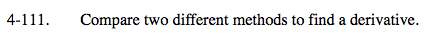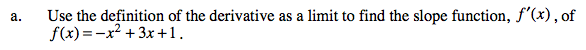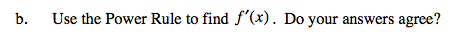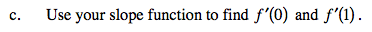### Home > CALC > Chapter 4 > Lesson 4.3.2 > Problem4-111

4-111.Substitute f(x) into the Definition of the Derivative (this is Hana's definition):

Find a way to 'cancel out the h' in the denominator:
1. Expand the numerator.
2. Combine like terms.
3. Factor out an h.
4. Cancel out the h.

Now that there is no longer an h in the denominator, take the limit as h→0.
This is the derivative of y = −x2 + 3x + 1.If your answers are different, then you probably made an algebraic error in part (a). Find it and fix it.f '(0) = 3, f '(1) = 1